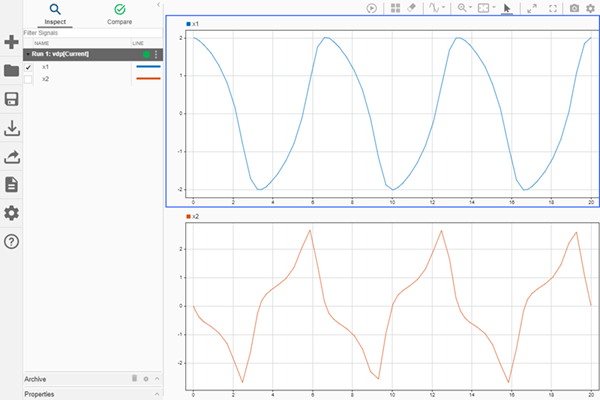Access data for a Simulation Data Inspector run

## Syntax

``run = Simulink.sdi.getRun(runID)``

## Description

example

````run = Simulink.sdi.getRun(runID)` returns a `Simulink.sdi.Run` object that provides access to the data in the run corresponding to the `runID`. The Simulation Data Inspector assigns run IDs when it creates a run. You can get the run ID for your run using `Simulink.sdi.getAllRunIDs` or `Simulink.sdi.getRunIDByIndex`.```

## Examples

collapse all

Many tasks performed using the Simulation Data Inspector programmatic interface start by accessing the `Simulink.sdi.Run` object that corresponds to the logged or imported data you want to analyze. For example, you can use the `Run` object to access the `Simulink.sdi.Signal` objects that correspond to individual signals in the run.

This example shows how to access `Run` objects by using the `Simulink.sdi.Run.getLatest` function, the `Simulink.sdi.getCurrentSimulationRun` function, or the `Simulink.sdi.getRun` function.

Create a Run

The model `sldemo_fuelsys` is already configured for logging. When you simulate the model, the Simulation Data Inspector automatically creates a run and assigns it a run ID.

```load_system('sldemo_fuelsys') sim('sldemo_fuelsys')```

Get Run Object Using `Simulink.sdi.Run.getLatest`

In this example, the run created when you simulated the model is the most recently created run in the Simulation Data Inspector. When you want to access the most recently created run, use the `Simulink.sdi.Run.getLatest` function.

`fuelsysRun = Simulink.sdi.Run.getLatest;`

Get Run Object Using `Simulink.sdi.getCurrentSimulationRun`

The run you want to access may not be the most recently created run in the Simulation Data Inspector. If the run corresponds to the most recent simulation of a model, you can use the `Simulink.sdi.getCurrentSimulationRun` function to access the `Run` object. You can also use the `Simulink.sdi.getCurrentSimulationRun` function to access data for an in-progress simulation when the simulation streams data to the Simulation Data Inspector. This function can be useful when you are working with multiple models.

In this example, the run created when you simulated the model is the current simulation run for the `sldemo_fuelsys` model.

`fuelsysRun = Simulink.sdi.getCurrentSimulationRun('sldemo_fuelsys');`

Get Run Object from a Run ID

When your task also requires the run ID, you can use the `Simulink.sdi.getRun` function to get the corresponding `Simulink.sdi.Run` object that contains the run data and metadata.

```runIDs = Simulink.sdi.getAllRunIDs; runID = runIDs(end); fuelsysRun = Simulink.sdi.getRun(runID);```

This example demonstrates how to access the `Simulink.sdi.Run` object for a Simulation Data Inspector run created by logging signals. From the `Simulink.sdi.Run` object you can get `Simulink.sdi.Signal` objects that contain the logged signal data and metadata. You can use the `Signal` objects and the `plotOnSubPlot` function to plot the data in the Simulation Data Inspector.

Create a Simulation Run and Access the `Run` Object

The `ex_vdp` model logs two signals. To create a simulation run containing the logged data, simulate the model.

`sim('ex_vdp');`

The Simulation Data Inspector keeps track of runs by assigning a unique numeric run ID to each run created by simulation, importing data, or opening a session. To access the run object for the simulation you just performed, use the `Simulink.sdi.getAllRunIDs` function and take the last run ID in the returned vector.

```runIDs = Simulink.sdi.getAllRunIDs; runID = runIDs(end);```

Once you have the run ID for the run, you can use the `Simulink.sdi.getRun` function to get the `Simulink.sdi.Run` object that corresponds to the run. You can use the `Run` object to check the metadata associated with the run, including the number of signals in the run.

```vdpRun = Simulink.sdi.getRun(runID); vdpRun.SignalCount```
```ans = int32 2 ```

Plot Data Using `Signal` Objects

Use the `getSignalByIndex` function to access signals from the `Run` object, `vdpRun`.

```signal1 = getSignalByIndex(vdpRun,1); signal2 = getSignalByIndex(vdpRun,2);```

Use the `Simulink.sdi.setSubPlotLayout` function to specify a 2-by-1 layout.

`Simulink.sdi.setSubPlotLayout(2,1)`

Before plotting the data, use the `Simulink.sdi.clearAllSubPlots` function to clear any data that is already plotted.

`Simulink.sdi.clearAllSubPlots`

Plot one signal on each subplot. To plot signals on the first subplot, you can set the `checked` property for the signal. To plot signals on subplots other than the first subplot, use the `plotOnSubPlot` function.

```signal1.Checked = true; plotOnSubPlot(signal2,2,1,true);```

View the Plotted Data

To view the plots you just created, open the Simulation Data Inspector using the `Simulink.sdi.view` function.## Input Arguments

collapse all

Run ID for the run you want a `Simulink.sdi.Run` object for. The Simulation Data Inspector assigns run IDs when it creates runs. You can get the run ID for a run using `Simulink.sdi.getAllRunIDs` or `Simulink.sdi.getRunIDByIndex`.

## Output Arguments

collapse all

`Simulink.sdi.Run` object for the run corresponding to the run ID.

## Version History

Introduced in R2011b